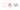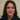# Javascript ES6 reduce() method and how to use it

We will talk about Javascript ES6 reduce() method and how to use it in this article. Among new ES6 features, reduce() method is one of the good and sometimes confusing ones. First we need to understand its syntax.

## The ES6 reduce() syntax

Basically reduce() is used when we need to sum up an arrays values and get a total amount at the end. Its callback function(the function to be applied by reduce) will execute on each element and takes up to four arguments that two of them are required for reduce to wrk. We call it a reduction. Here is the syntax:

`<i>array</i>.<span class="color_h1">reduce</span>(<em>function(total, currentValue, currentIndex, arr), initialValue</em>);`

The total value is the initial value or returned value of the function. It is the first value of the array that we do the reduction for. We will explain it later.

The currentValue is the value of the current element. It is the right hand side of the latest element.

These two values are required for reduce to work. Time for some examples.

### A full example

This is an ES6 reduce example:

`[0, 1, 2, 3, 4].reduce(function(accumulator, currentValue, currentIndex, array) {`
`return accumulator + currentValue;`
`});`

We have applied reduce to an array of five elements. In the below table you will see what happens to all those elements inside the function:

callback accumulator currentValue currentIndex array return value
first call 1 1 [0, 1, 2, 3, 4] 1
second call 1 2 2 [0, 1, 2, 3, 4] 3
third call 3 3 3 [0, 1, 2, 3, 4] 6
fourth call 6 4 4 [0, 1, 2, 3, 4] 10

Clearly the accumulator which is called the total amount as well, is always the left value in the array and the current value is in the right side of it. Remember reduce applies he function to each element in the array so these values are changing upon each call until it reaches the last value.

And the best thing is to convert this to an arrow function syntax. We leave off the currentIndex and array as they are optional:

`[0, 1, 2, 3, 4].reduce((accumulator, currentValue)=> accumulator + currentValue);`

### Initial value

If we were to have an initial value which we might incases, the code will change to this:

`[0, 1, 2, 3, 4].reduce((accumulator, currentValue, currentIndex, array) =>``accumulator + currentValue``, 10);`

And the reduction will add up by the initial value and in this case 10. So the output is 20 instead of 10 in the above example.

### An example with objects

We could apply reduce to objects in a similar away:

`var initialValue = 0;`
`var sum = [{x: 1}, {x:2}, {x:3}].reduce( (accumulator, currentValue) => accumulator + currentValue.x`
`,initialValue`
`);`

`console.log(sum) // logs 6`

Which accumulates the object values and print them out.

### Counting instances of values in an object {#Countinginstancesofvaluesinanobject.highlight-spanned}

In the example below we want to count how many times a name has been repeated inside the names array. If the name was repeated meaning the current value (name) was the same as total value (allNames) , we increment the total count by one and if not, we just count it as one repeat:

`var names = ['Alice', 'Bob', 'Tiff', 'Bruce', 'Alice'];`

`var countedNames = names.reduce(function (allNames, name) {`
`if (name in allNames) {`
`allNames[name]++;`
`}`
`else {`
`allNames[name] = 1;`
`}`
`return allNames;`
`}, {});`
`// countedNames is:`
`// { 'Alice': 2, 'Bob': 1, 'Tiff': 1, 'Bruce': 1 }`

There are lots of different use cases for reduce as it is a very useful and multipurpose method. We have shown a few of them here. In the upcoming articles, we will challenge ourselves in some difficult examples around reduce and other ES6 array methods. Thanks for reading.

© 2020This site is created and maintined by Azadeh Faramarzi , A passionate developer, sport and code lover who loves to share and write when she has some time.